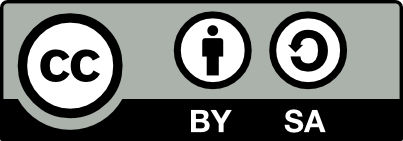Journal DetailsFormat
Journal
eISSN
1898-9934
ISSN
1426-2630
First Published
09 Jun 2008
Publication timeframe
4 times per year
Languages
English
Open Access

# Introduction to Stopping Time in Stochastic Finance Theory

###### Received: 27 Jun 2017
Journal DetailsFormat
Journal
eISSN
1898-9934
ISSN
1426-2630
First Published
09 Jun 2008
Publication timeframe
4 times per year
Languages
English

We start with the definition of stopping time according to , p.283. We prove, that different definitions for stopping time can coincide. We give examples of stopping time using constant-functions or functions defined with the operator max or min (defined in , pp.37–38). Finally we give an example with some given filtration. Stopping time is very important for stochastic finance. A stopping time is the moment, where a certain event occurs (, p.372) and can be used together with stochastic processes (, p.283). Look at the following example: we install a function ST: {1,2,3,4} → {0, 1, 2} ∪ {+∞}, we define:

a. ST(1)=1, ST(2)=1, ST(3)=2, ST(4)=2.

b. The set {0,1,2} consists of time points: 0=now,1=tomorrow,2=the day after tomorrow.

We can prove:

c. {w, where w is Element of Ω: ST.w=0}=∅ & {w, where w is Element of Ω: ST.w=1}={1,2} & {w, where w is Element of Ω: ST.w=2}={3,4} and

ST is a stopping time.

We use a function Filt as Filtration of {0,1,2}, Σ where Filt(0)=Ωnow, Filt(1)=Ωfut1 and Filt(2)=Ωfut2. From a., b. and c. we know that:

d. {w, where w is Element of Ω: ST.w=0} in Ωnow and

{w, where w is Element of Ω: ST.w=1} in Ωfut1 and

{w, where w is Element of Ω: ST.w=2} in Ωfut2.

The sets in d. are events, which occur at the time points 0(=now), 1(=tomorrow) or 2(=the day after tomorrow), see also , p.371. Suppose we have ST(1)=+∞, then this means that for 1 the corresponding event never occurs.

As an interpretation for our installed functions consider the given adapted stochastic process in the article .

ST(1)=1 means, that the given element 1 in {1,2,3,4} is stopped in 1 (=tomorrow). That tells us, that we have to look at the value f2(1) which is equal to 80. The same argumentation can be applied for the element 2 in {1,2,3,4}.

ST(3)=2 means, that the given element 3 in {1,2,3,4} is stopped in 2 (=the day after tomorrow). That tells us, that we have to look at the value f3(3) which is equal to 100.

ST(4)=2 means, that the given element 4 in {1,2,3,4} is stopped in 2 (=the day after tomorrow). That tells us, that we have to look at the value f3(4) which is equal to 120.

In the real world, these functions can be used for questions like: when does the share price exceed a certain limit? (see , p.372).

#### MSC 2010

 Grzegorz Bancerek. The fundamental properties of natural numbers. Formalized Mathematics, 1(1):41–46, 1990.Search in Google Scholar

 Czesław Byliński. Functions and their basic properties. Formalized Mathematics, 1(1): 55–65, 1990.Search in Google Scholar

 Czesław Byliński. Functions from a set to a set. Formalized Mathematics, 1(1):153–164, 1990.Search in Google Scholar

 Hans Föllmer and Alexander Schied. Stochastic Finance: An Introduction in Discrete Time, volume 27 of Studies in Mathematics. de Gruyter, Berlin, 2nd edition, 2004.10.1515/9783110212075Search in Google Scholar

 Peter Jaeger. Modelling real world using stochastic processes and filtration. Formalized Mathematics, 24(1):1–16, 2016. doi: 10.1515/forma-2016-0001.10.1515/forma-2016-0001

 Achim Klenke. Wahrscheinlichkeitstheorie. Springer-Verlag, Berlin, Heidelberg, 2006.Search in Google Scholar

 Jürgen Kremer. Einführung in die diskrete Finanzmathematik. Springer-Verlag, Berlin, Heidelberg, New York, 2006.Search in Google Scholar

 Andrzej Nędzusiak. σ-fields and probability. Formalized Mathematics, 1(2):401–407, 1990.Search in Google Scholar

 Andrzej Trybulec. Binary operations applied to functions. Formalized Mathematics, 1(2): 329–334, 1990.Search in Google Scholar

• #### The 3-Fold Product Space of Real Normed Spaces and its Properties

Recommended articles from Trend MD